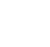# The basics of understanding financial statements

Monitor the financial information submitted by your accountants
By Henry Ong |

An entrepreneur needs to develop some sense of control the company finances. One form of control you can exercise is to analyze the financial information generated by your accounting system. The data in your financial statements can regularly provide you with valuable signals and insights about where your business is heading.

Precisely how do you analyze financial information?

Ratio analysis. A common way to analyze a financial statement is by computing ratios. When you do a ratio analysis, you try to extract a meaningful relationship between any two numbers in a financial statement. This helps you identify potential problem areas and opportunities within the company.

You need not let the details of your financial statement overwhelm you, and you need not take so many ratios either. In practice, you can adequately see your financial position by taking just three sets of ratios: the liquidity ratios, the leverage ratios, and the profitability ratios.

Liquidity ratios. The ability of your business to pay its short-term suppliers can be measured by the current ratio. The current ratio, which belongs to a bigger class of liquidity ratios, is the result when you divide current assets by current liabilities. Current assets are those assets that your business expects to convert into cash within the year, such as cash, accounts receivable, and inventory. Current liabilities, on the other hand, are those financial obligations that you expect to pay within the year, such as accounts payable and accruals.

For example, assume that your balance sheet gives you the following information:

Current assets: P150,000
Current liabilities: P100,000
Current ratio: P150,000 ÷ P100,000
= 1.5

This means that for every P1.50 worth of cash, receivables, and inventory in your current assets, you have P1.00 worth of payables. This may seem good at first, but as a rule, a current ratio of at least 2 is preferable. This means that for every peso that you need to pay, you have P2 in store or in your current assets.

Generally, the higher the current ratio is, the stronger the financial position of your company. This, however, is not an indication that you are managing your resources efficiently. For example, you may have accumulated so much cash in your bank account, thus increasing your current ratio. This may mean that you may be very financially liquid—you have so much cash—but you may not be maximizing your opportunities to earn. Indeed, you could have invested that extra cash in another business or in a high interest-bearing money-market investment.

Leverage ratios. After evaluating liquidity, you need to analyze the extent to which your business relies on other people’s money to finance its investments and operations. The leverage ratios are your tools for doing this type of analysis.

The leverage ratio measures the degree of financial risk you are taking in doing your business. The higher the risk is, the greater the probability of huge losses if your sales projections are not achieved.

One example of leverage ratio is the debt ratio, which measures the percentage of your debt in relation to your total assets.

To illustrate, take the following example:

Total debt: P750,000
Total assets: P1,000,000
Debt ratio: P750,000 ÷ P1,000,000
= 0.75 or 75 percent

You can also compute your debt-to-equity ratio by first subtracting total debt from total assets to get your total equity (or that amount which you own, and do not owe others), and then dividing your total debt by your total equity.

Using the previous example:

Total equity: P1,000,000 - P750,000
= P250,000
Debt equity: P750,000 ÷ P250,000
= 3.

This means that for every P3 you borrowed to finance your business, you have put in P1 worth of your personal capital.

As a rule, you should not borrow more than half of your total assets, which means having a debt ratio less than 50 percent.

Financial ratios are useful tools for evaluating business performance. However, ratios alone are not enough. You need to know which ratio is most crucial to your business, after which you should focus on it to achieve your financial objectives.

Close